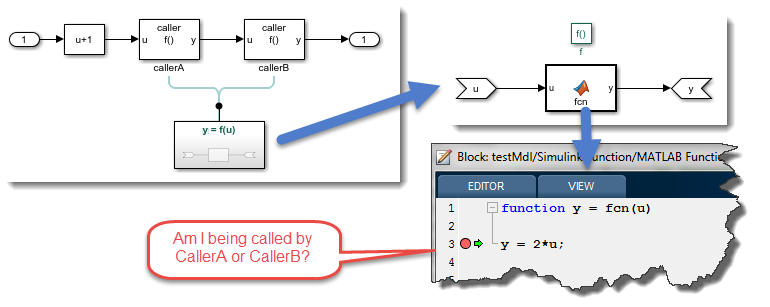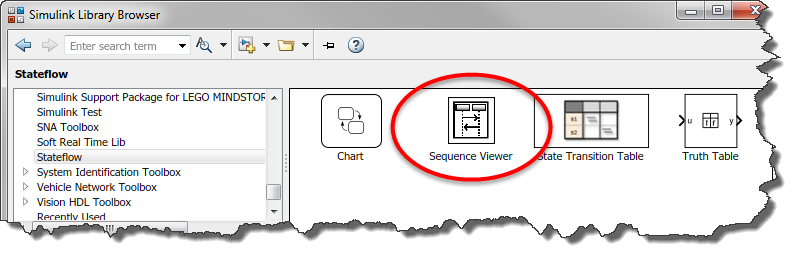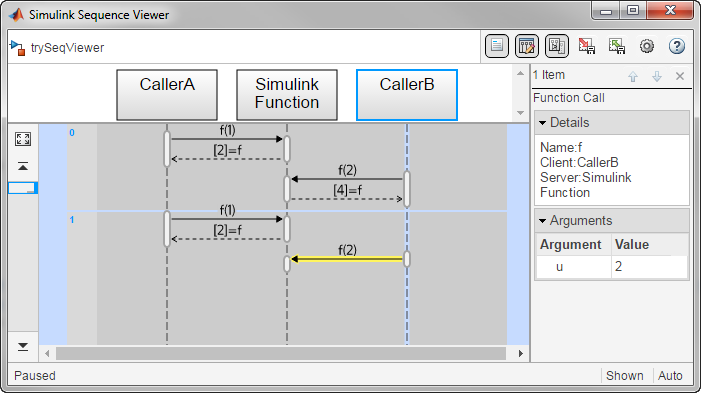Who’s Calling?

Did you know that it is possible to visualize the calling of Simulink Functions using the Sequence Viewer? Let's see how that works and in what type of situations it can be particularly useful.

The Question

Here is a question I have recently been asked:

I have a Simulink model with a Simulink Function. The model contains multiple Function CAller blocks that can call this Simulink Function. The Simulink Function contains a MATLAB Function block. When I put a breakpoint in the MATLAB Code, I want to know from which Function Caller block originated the call.The Sequence Viewer

The answer is a feature I typically use when dealing with SimEvents and Stateflow: The Sequence Viewer.When the breakpoint in the MATLAB Function block will be hit, you will know that the last client in the list is the one calling. In this image, we are at simulation time t=1sec, and the client is CallerB: